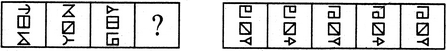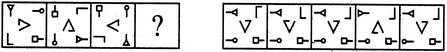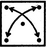# Non Verbal Reasoning - Analogy

### Exercise :: Analogy - Section 2

Each of the following questions consists of two sets of figures. Figures A, B, C and D constitute the Problem Set while figures 1, 2, 3, 4 and 5 constitute the Answer Set. There is a definite relationship between figures A and B. Establish a similar relationship between figures C and D by selecting a suitable figure from the Answer Set that would replace the question mark (?) in fig. (D).

46.

Select a suitable figure from the Answer Figures that would replace the question mark (?).(A)     (B)      (C)     (D)                  (1)      (2)      (3)      (4)      (5)

 A. 1 B. 2 C. 3 D. 4 E. 5

Explanation:

The upper and the lower elements interchange places; the element that reaches the upper position gets laterally inverted; the element that reaches the lower position gets laterally inverted and its head also gets laterally inverted; the line segment inside the square (central element) rotates 45oCW.

47.

Select a suitable figure from the Answer Figures that would replace the question mark (?).(A)     (B)      (C)     (D)                  (1)      (2)      (3)      (4)      (5)

 A. 1 B. 2 C. 3 D. 4 E. 5

The elements move in the sequencethe elements reaching the upper-left and the lower-right positions rotate 90oACW; the elements reaching the upper-right and lower-left positions rotate 90oCW; the central element rotates 90oACW and gets laterally inverted.